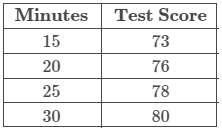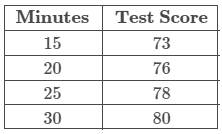Question

Janet is studying the relationship between the average number of minutes spent exercising per day and math test scores and has collected the data shown in the table. The line of best fit for the data is yˆ=0.46x+66.4. Assume the line of best fit is significant and there is a strong linear relationship between the variables.(a) According to the line of best fit, what would be the predicted test score for someone who spent 23 minutes exercising? Round your answer to two decimal places.

The predicted test score is 76.98

Question

Janet is studying the relationship between the average number of minutes spent exercising per day and math test scores and has collected the data shown in the table. The line of best fit for the data is yˆ=0.46x+66.4.(a) According to the line of best fit, the predicted test score for someone who spent 23 minutes exercising is 76.98.

(b) Is it reasonable to use this line of best fit to make the above prediction?